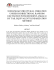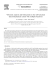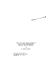Model Reduction and Controller Design for a Nonlinear Heat

Transcription

Model Reduction and Controller Design for a Nonlinear Heat
```International Journal of Automation and Computing
9(5), October 2012, 474-479
DOI: 10.1007/s11633-012-0669-6
Model Reduction and Controller Design for a Nonlinear
Heat Conduction Problem Using Finite Element Method
Paramita Guha
Mashuq Un Nabi
Department of Electrical Engineering, Indian Institute of Technology, Delhi 110016, India
Abstract: The mathematical models for dynamic distributed parameter systems are given by systems of partial differential equations.
With nonlinear material properties, the corresponding finite element (FE) models are large systems of nonlinear ordinary differential
equations. However, in most cases, the actual dynamics of interest involve only a few of the variables, for which model reduction
strategies based on system theoretical concepts can be immensely useful. This paper considers the problem of controlling a three
dimensional profile on nontrivial geometries. Dynamic model is obtained by discretizing the domain using FE method. A nonlinear
control law is proposed which transfers any arbitrary initial temperature profile to another arbitrary desired one. The large dynamic
model is reduced using proper orthogonal decomposition (POD). Finally, the stability of the control law is proved through Lyapunov
analysis. Results of numerical implementation are presented and possible further extensions are identified.
Keywords:
Thermal systems, partial differential equations, finite element (FE) models, model reduction techniques, proper orthogonal decomposition (POD), nonlinear control, Lyapunov stability.
1
Introduction
In many engineering applications, like material processing, hardening, etc., the temperature profile over a workpiece or assembly has to be controlled over a span of time.
Most of such thermal systems belong to a class of “distributed parameter system”, which are governed by partial
differential equations and are infinite-dimensional in nature.
Control of such systems through analytical approaches have
been studied , but are mostly for simple geometries. As
an alternative, numerical methods like finite difference (FD)
and finite element (FE)[2, 3] , methods can be used for modeling, analysis, and control purposes.
This paper presents a nonlinear modeling and control
strategy for a heating problem with an arbitrary shape
and nonlinear thermal conductivity. The partial differential
equation model is discretized using FE method, and a nonlinear ordinary differential equation model is obtained. This
model is reduced using proper orthogonal decomposition .
Finally, a nonlinear control law is proposed for transferring
the temperature distribution over a three dimensional domain from any arbitrary initial profile to any desired one.
Such control problems can often occur in heat treatment
processes. Thus, a state feedback controller is designed for
the temperature control, output feedback can also be designed as given in [5, 6].
For the proposed control law, the convergence of the temperature profile to the desired profile is established by a
Lyapunov criteria. Numerical results along with conclusions are presented.
This work can be considered as an extension of our previous work . In that work, a two dimensional geometry
is considered, for which a modeling and a nonlinear control
strategy are proposed. The paper is organized as follows.
In Section 2, the basic concepts behind the modeling and
model reduction of a system in general are described. The
Manuscript received July 14, 2011; revised March 13, 2012
physical problem considered and its reduced order modeling are discussed in Sections 3 and 4, respectively. Based
on the reduced model, a controller is proposed in Section 5.
Results of numerical simulations are presented in Section 6.
The conclusions are drawn in Section 7.
2
Modeling and model reduction
Modeling plays a very important role in the quantitative
analysis of a system. A mathematical model in many cases
is considered as the most valuable means to obtain inputoutput relationship. But, obtaining a mathematical model
is often very difficult and costly, especially for many practical systems. Generally, most practical systems (e.g., electromagnetic, mechanical, thermal, electromechanical, etc.)
are governed by partial differential equations (PDEs). Obtaining simple input-output relationships for these systems
is very difficult. In order to reduce the mathematical complexities, we need to convert the PDEs to simple ordinary
differential equations (ODEs). Now, these sets of equations
can be handled quite easily. There are several mathematical tools available for the purpose of obtaining ODEs from
PDEs, e.g., finite element method (FEM), finite difference
method (FDM), etc.
The only disadvantage in this approach is that the number of variables as well as the size of the ODE system
increases massively. In order to analyze the system, the
size of the model must be reduced. So, model reduction is
necessary[8, 9] . There are various methods for this purpose,
like proper orthogonal decomposition (POD), Krylov subspace method, modal decomposition method, etc. The aim
of model reduction is to reduce the size of the system that
the dynamic characteristics of both original and reduced
models remain almost the same. The model reduction techniques can be broadly classified into two categories:
1) Gramian based method (singular value decomposition): POD method, Hankel singular value decomposition
and approximation by balanced truncation.
475
P. Guha and M. U. Nabi / Model Reduction and Controller Design for a Nonlinear · · ·
2) Moment matching based method (Krylov): Arnoldi
method, Lanczos method and Rational Krylov.
POD method is mostly used to reduce of size of nonlinear
systems[10−12] . Here, a smaller subspace is obtained which
corresponds to the approximated controllability subspace
of the original model. The controllability subspace of the
model is obtained by simulating the original model for some
given excitations. Now, the state space of the given model
is projected on this subspace. This yields a fairly accurate
but still nonlinear reduced model. This method is popularly
used for various nonlinear systems like electromagnetic systems, chemical reactors etc.[13, 14]
Another popular method mostly used for linear systems
is Krylov subspace method[15, 16] . Here, the basis for the
reduced system can be obtained by considering a few moments of the original nonlinear model. A reduced model is
constructed which has certain number of moments of the
same value as the original one. The main advantage of
this method is that the sparsity is preserved in the reduced
model. This gives a considerably accurate reduced order
model with relatively greater numerical stability, and memory required for storage purpose is very less . However,
the asymptotic stability of the model cannot be guaranteed.
This method has been generally used in power systems .
3
Problem formulation
For the present paper, we have considered a typical three
dimensional heating problem as shown in Fig. 1.
This can be considered as a section of railway track bullhead.
Here, any convective and radiative heat transfer is neglected for the sake of simplicity. In addition, the input
f = 0 is chosen as there is no body heating. In control engineering, heat equations involving control inputs applied at
the boundaries, have been an important subject of study .
Here, constant Dirichlet temperature conditions Td are applied at the bottom regions. A heat flux input q(t) is applied on the top as a Neumann condition which acts as a
control input, as shown in Fig. 1. The suggested methodology can be applied for time varying Dirichlet conditions as
well. The work piece is made of carbon steel and its thermal conductivity is nonlinearly dependent on temperature.
Here, we have taken thermal conductivity as proportional
to T 4 . This makes the entire system nonlinear. Now, the
governing equation can be written as
c1
∂T
+ ∇(κ(T )∇T ) = 0
∂t
(2)
where c1 = cρ and κ(T ) ∝ T 4 . The boundary conditions
can be expressed as
Γq :
∂T
= q(t),
∂n
Γd : T = Td .
Obtaining the temperature profiles from (2) is quite difficult. Hence, a numerical approach is necessary. Here, we
have applied FEM for this purpose. The corresponding finite element (FE) model can be written as
∂ T̄
+ K(T̄ )T̄ = b̄q(t) − T̄d
∂t
(3)
where T̄ is the vector of nodal temperatures, K(T̄ ) is the
coefficient matrix, T̄d is a vector related to Td , and the
constant c1 is absorbed into the matrices. Finally, q(t) is
the control input and b̄ is the input vector related to q(t).
4
Fig. 1
As discussed earlier, the size of the ODE model increases
massively after discretization. This large size is quite inconvenient for further analysis. Hence, model reduction is
necessary. While reducing, it should be kept in mind that
the dynamic properties of both the reduced and original
models remain almost same.
To construct the POD model, the heat equation is simulated for a given excitation, and the time-response states
are accumulated as columns of “snapshot” matrix S m×n .
Here, m denotes the number of states of the original model,
and n is the number of snapshots. Let the singular value
decomposition (SVD) of S be given by
Three dimensional problem domain
The governing equation for conductive heat flow over the
3D domain can be written as
∂T (x, y, z, t)
+ ∇(κ∇T (x, y, z, t)) = f
cρ
∂t
Reduced order modeling
(1)
where c, ρ, and κ denote the specific heat, density, and thermal conductivity of the concerned material, respectively.
Here, T (x, y, z, t) represents time-dependent temperature in
Cartesian coordinate system of the domain. The right hand
side vector f denotes any heat source inside the domain.
U T SV = Σ
where Σm×n is a diagonal matrix of size m × n, and its
elements are the singular values of S arranged in decreasing order. The magnitude of a singular value indicates the
participation strength of corresponding “mode” in the final
solution.
Now, in problems like the one considered here, owing to
poor controllability, the actual range of the snapshot matrix
is much smaller compared to the space state model. Hence,
476
International Journal of Automation and Computing 9(5), October 2012
only a first few, say r, singular values are significant and
dominant. Therefore, we can write
taken P as an identity matrix for the sake of simplicity.
Hence, (8) can be written as
Σ = diag{[Σr , 0]}.
φ=
The range space of the snapshot matrix can be obtained
by considering only first r columns of U m×m , i.e., Ur =
[u1 , u2 , · · · , ur ]. Further, the original nonlinear model can
be projected on subspace Ur . The transformation equation
can now be written as
T̄ = Ur z
ż(t) = −K̃z(t) − T̃d + b̃q(t)
(5)
where K̃ = UrT KUr , T̃d = UrT T̄d , and b̃ = UrT b̄. Like K, K̃
is also dependent on temperature T̄ , hence, the nonlinearity
is also preserved in the reduced model.
5
As mentioned earlier, the aim of the controller design
problem is to heat the domain from any given initial temperature profile to any arbitrary desired temperature profile. Let T̄0 and T̄ ∗ denote the initial and final desired
temperature profiles, respectively. If both T̄0 and T̄ ∗ represent physically meaningful and occurring temperature profiles, then they can be expected to lie predominantly in the
span of Ur , and the range space of the snapshot matrix S.
Then, the corresponding reduced states z0 and z ∗ will satisfy T̄0 = Ur z0 and T̄ ∗ = Ur z ∗ . Hence, it can be seen that
the problem of transferring T̄0 to T̄ ∗ becomes equivalent to
transferring the reduced state z from z0 to z ∗ . On the other
hand, if T0 or T ∗ does not lie in the span of Ur , z0 and z ∗
can be taken as their respective least square approximations
in the projected subspace, i.e., span of Ur .
Now, in order to transfer the reduced state z of the reduced system (5) from z0 to z ∗ using the control input q(t),
the following control law is proposed.
q(t) =
1
· eT (K̃z ∗ + T̃d ).
eT b̃
(6)
The above control law takes z from the current z0 to the
desired z ∗ . The corresponding error between the two states
can be given as
e(t) = z(t) − z ∗ (t).
Hence, substituting this in (5), the dynamics of this error
are obtained as
∗
ė(t) = −K̃(e + z (t)) − T̃d + b̃q(t).
(7)
In order to show that the above error settles to zero, we
define a Lyapunov function as
φ=
1 T
e Pe
2
φ̇ = eT ė =
eT {−K̃(e + z ∗ (t)) − T̃d + b̃q(t)} =
−eT K̃(e + z ∗ (t)) − eT T̃d +
1
eT b̃ · {
· eT (K̃z ∗ + T̃d )} =
(eT b̃)
−eT K̃e 6 0.
Since K̃ is positive definite, the origin is a stable equilibrium
point for the nonlinear error dynamics (7). Hence, e = z−z ∗
settles to zero, and equivalently z(t) settles to z ∗ as desired,
guaranteing the temperature profile to settle at T ∗ .
6
6.1
Controller design
(8)
where P is a positive definite matrix, making the Lyapunov
candidate function φ also positive definite. Here, we have
(9)
Now, using (9), (7), and (6) successively, we can have
(4)
where z r×1 are the states of the reduced system. Using
the above transformation in (3), a reduced model can be
obtained as
1 T
e e.
2
Numerical results
Generation of reduced order model
The proposed methodology is applied on the three dimensional geometry shown in Fig. 1. The problem domain
is discretized into tetrahedral FE mesh with 2754 nodes excluding the Dirichlet boundary. Therefore, the corresponding FE model for the temperature dynamics is a system
of 2754 nonlinear equations as given by (3). To generate
the reduced model, the original model is simulated with an
impulse input. Here, in order to capture the widest frequency characteristics, the input is taken as impulse input.
For simulations, backward difference is used, with 30 time
steps each of 1 s.
At each time-step, matrix K is dependent on the temperature, and the resulting nonlinear algebraic equation system
is solved using the Newton-Raphson method. Finally, the
30 temperature profiles for the time-steps or temperature
“snapshots” are stored as columns of matrix S. The rank
of the snapshot matrix is found as 20, beyond which the
magnitudes of the singular values are negligible. This leads
to the reduction of original nonlinear model to nonlinear
models of the form (5) but with a much reduced size of 20.
As a validation, the reduced model (5) was simulated with
q(t) as an impulse δ(t), and the nodal temperature profiles
were recomputed using (4). The temperature profiles were
found to agree satisfactorily with those computed originally
from the unreduced model (3). It has been observed that
at t = 30 s, the error is around 1.05%. This justifies the
reduced model for the considered problem.
6.2
Application of control law
The proposed control law is first tested to simulate the
reduced model (5). It is then combined with (6) to steer
the current state z0 to a arbitrary desired state z ∗ . As
established theoretically, in each case, the reduced model
converges to the desired z ∗ . An example case is where z0 is
chosen arbitrarily while the target z ∗ is chosen to have all
its components as 1050.
Next, the control law is tested by applying the same to
the original full-size FE model. It implies that the control
P. Guha and M. U. Nabi / Model Reduction and Controller Design for a Nonlinear · · ·
input will be applied to the actual physical system with
temperature variable T rather than the mathematical variable z. This constitutes a more complete numerical validation of the control law. For this, the two following different
numerical simulations are considered.
Simulation 1. The initial temperature profile of the
bullhead is taken to be 27o C uniformly over the body, as
shown in Fig. 2. The target or desired temperature profile is
taken to be as shown in Fig. 3. When the proposed control
law is applied as the heat input, the time evolution of the
temperature profile is shown as snapshots at t = 10 s, t =
20 s and at t = 30 s, in Figs. 4–6 respectively. By comparing
Fig. 6 with Fig. 3, it can be clearly seen that at t = 30 s, the
actual temperature profile of the bullhead has reached to
almost exactly the desired temperature profile.
Simulation 2. In this case, the initial temperature profile is taken to be the same as before, i.e., uniform temperature of 27o C, while the target temperature profile is taken
to be the one as shown in Fig. 7. In this case, the time evolution of the temperature profile as a result of the control
heat input is shown at t = 10 s, 20 s, and 30 s in Figs. 8–10.
Also, by comparing Figs. 7 and 10, it can be seen that the
temperature profile reaches its desired nature within 30 s of
applying the control heat input.
Finally, referring to the control law (6), it may be noted
that the control expression involves quantities from the reduced model of size r. Hence, it can be concluded that only
20 variables are sufficient to represent the original model of
size 2754.
7
477
interesting and will be investigated further.
Fig. 2
etry
Initial temperature, t = 0 s profile over the entire geom-
Conclusions
In this paper, we have considered modeling and control of
a thermal problem over a three dimensional geometry where
the temperature is increased from any arbitrary profile to a
desired value. The method is based on FEM, so the above
scheme can be applied for any complex shaped geometry. A
reduced order dynamic model is obtained by applying the
POD method. It has been observed that when represented
in full coordinates, the error between the outputs of both
models is very small. A control strategy is presented and
applied on the reduced model, which transfers the initial
temperature profile to a desired one. The convergence of
the resulting feedback system to the desired temperature
profile is proved through Lyapunov criteria, and simulation
results are presented. The error between the temperature
profile of the unreduced system with the controller and the
desired temperature profile T ∗ are quite small. So, it can
be concluded that the control law works quite well on the
model. This leads to the concept of a closed loop observer,
by which we observe the outputs and they are fed back to
the original system to get the desired output. This work
can be considered as the basis for a more comprehensive
work towards the development of control strategies for such
systems. Also, this may be useful for simple modeling of
distributed parameter systems. In the present work, for
the sake of simplicity, we have neglected non-conductive
heat transfers, e.g., radiative, convective, etc. These can
be included to make the problem more realistic. Also, the
effects of error occurring due to the model reduction process
and parameter uncertainties in modeling of the system are
Fig. 3 Simulation 1: Desired temperature profile over the entire
geometry
Fig. 4
10 s
Simulation 1: Temperature profile with controller at t =
478
International Journal of Automation and Computing 9(5), October 2012
Fig. 5
20 s
Simulation 1: Temperature profile with controller at t =
Fig. 6
30 s
Simulation 1: Temperature profile with controller at t =
Fig. 7 Simulation 2: Desired temperature profile over the entire
geometry
Fig. 8
10 s
Simulation 2: Temperature profile with controller at t =
Fig. 9
20 s
Simulation 2: Temperature profile with controller at t =
Fig. 10
30 s
Simulation 2: Temperature profile with controller at t =
P. Guha and M. U. Nabi / Model Reduction and Controller Design for a Nonlinear · · ·
References
 B. Bamieh. The structure of optimal controllers of spatiallyinvariant distributed parameter systems. In Proceedings of
the 36th Conference on Decision and Control, IEEE, San
Diego, USA, pp. 1056–1061, 1997.
 J. N. Reddy. An Introduction to the Finite Element
Method. 2nd ed., New York, USA: McGraw-Hill Science,
1993.
 M. V. K. Chari, S. J. Salon. Numerical Methods in Electromagnetism. New York, USA: Academic Press, 2000.
 A. Armaou, P. D. Christofides. Dynamic optimization of
dissipative PDE systems using empirical eigenfunctions. In
Proceedings of the American Control Conference, IEEE,
USA, Anchorage AK, USA, vol. 2, pp. 1040–1048, 2002.
 N. Duan, F. N. Hu, X. Yu. An improved control algorithm
for high-order nonlinear systems with unmodelled dynamics. International Journal of Automation and Computing,
vol. 6, no. 3, pp. 234–239, 2009.
 K. Alimhan, H. Inaba. Output feedback control for a class
of nonlinear systems. International Journal of Automation
and Computing, vol. 3, no. 3, pp. 215–221, 2006.
 M. Nabi, P. Guha. A control law for a nonlinear heat conduction problem on nontrivial domains using FEM. In Proceedings of the 17th Mediterranean Conference on Control
and Automation, IEEE, Washington, DC, USA, pp. 320–
323, 2009.
 R. F. Curtain. Model reduction for control design for
distributed parameter systems. In Research Directions in
Distributed Parameter Systems, SIAM, Philadelphia, PA,
pp. 95–121, 2003.
 E. B. Rudnyi, J. G. Korvink. Model order reduction for
large scale finite element engineering models. In Proceedings of European Conference on Computational Fluid Dynamics, Delft, The Netherlands, 2006.
 A. Chatterjee. An introduction to the proper orthogonal
decomposition. Current Science, vol. 78, no. 7, pp. 808–817,
2000.
 C. W. Rowley. Model reduction for fluids, using balanced
proper orthogonal decomposition. International Journal of
Bifurcation and Chaos, vol. 15, no. 3, pp. 997–1013, 2005.
 J. R. Singler. Approximate low rank solutions of Lyapunov
equations via proper orthogonal decomposition. In Proceedings of American Control Conference, IEEE, Seattle, USA,
pp. 267–272, 2008.
 Y. Zhai, L. Vu-Quoc. Analysis of power magnetic components with nonlinear static hysteresis: Proper orthogonal
decomposition and model reduction. IEEE Transactions on
Magnetics, vol. 33, no. 5, pp. 1888–1897, 2007.
479
 M. A. Singer, W. H. Green. Using adaptive proper orthogonal decomposition to solve the reaction-diffusion equation.
Applied Numerical Mathematics, vol. 59, no. 2, pp. 272–279,
2009.
 R. W. Freund. Krylov-subspace methods for reduced-order
modeling in circuit simulation. Journal for Computational
and Applied Mathematics, vol. 123, no. 1–2, pp. 395–421,
2000.
 B. N. Datta. Krylov subspace methods for large-scale matrix problems in control. Future Generation Computer Systems, vol. 19, no. 7, pp. 1253–1263, 2003.
 E. J. Grimme. Krylov Projection Methods for Model Reduction, Ph. D. dissertation, University of Illinios at Urbana
Champaign, Urbana IL, USA, 1997.
 D. Chaniotis, M. A. Pai. Model reduction in power systems using Krylov subspace methods. IEEE Transactions
on Power Systems, vol. 20, no. 2, pp. 888–894, 2006.
 D. M. Bos̆sković, M. Krstić W. Liu. Boundary control of
an unstable heat equation via measurement of domainaveraged temperature. IEEE Transactions on Automatic
Control, vol. 46, no. 12, pp. 2022–2028, 2001.
Paramita Guha graduated from Jalpaiguri Goverment Engineering College, India
in 2001. She received the M. Eng. degree
from Bengal Engineering College (Deemed
University), India in 2003. She is currently
a Ph. D. candidate in electrical engineering
from Indian Institute of Technology (IIT),
Delhi, India.
Her research interests include distributed
parameter systems, coupled systems, modeling and simulation, model reduction and control theory.
E-mail: [email protected] (Corresponding author)
Mashuq Un Nabi graduated from Jadavpur University (JU), India in 1997. He
received the M. Tech degree from IIT Kanpur, India in 1999 and Ph. D. degree from
IIT Bombay, India in 2004. He is currently
an assistant professor in the Department of
Electrical Engineering in IIT Delhi, India.
His research interests include computational algorithms for control systems, distributed systems modeled by partial differential equations (PDEs), and electromagnetic fields.
E-mail: [email protected]
```

Parametric and State Estimation of Nonlinear Systems 15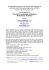OGT Review Worksheet Patterns, Functions, and Algebra – Part One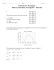Nonlinear MPC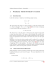portable usb dj mixer + scratch controll er + dj software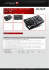PrimeFilm 1800 AFL Low-Cost 35mm Slide/Film Scanning Through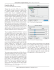Active Control of Separated FlowThe new RS-35M, June, 2010 - Stu Martin, [email protected]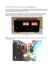adaptive nonlinear control of loudspeaker systems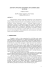BALTICA 10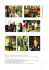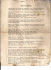Статья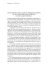Ripples, Twisters and Avalanches in Plasma Phase Space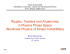Updating Frequency Response Functions of Linear Structures With Localized Nonlinearities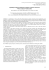Numerical solution of nonlinear system of parial differential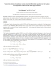2008 Suzuki GSXR750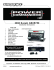SISTEM INFORMASI MANAJEMEN - Blogs at Universitas Budi Luhur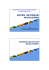Improved Sample Complexity Estimates for Statistical Learning Control of Uncertain Systems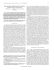Participation of Project Leader in Scientific meetings in 2014.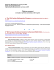Haunch preparation - The Deer Initiative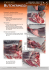telm